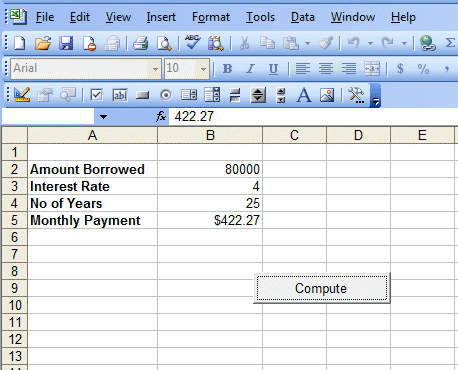Creating a Financial Calculator

[Back to Excel VBA Sample Code]

We can write Excel VBA code to perform complex financial calculations. Here we have created a financial calculator to calculate the periodic payment for a loan taken from the bank. Below is the Excel VBA code for the financial calculator and its output interface.

 Private Sub CommandButton1_Click() Dim N As Integer Dim p, pmt, rate, I, PVIFA As Double p = Cells(2, 2) rate = Cells(3, 2) N = Cells(4, 2) * 12 I = (rate / 100) / 12 PVIFA = 1 / I - 1 / (I * (1 + I) ^ N) pmt = p / PVIFA Cells(5, 2) = Format(pmt, "\$#,##0.00") End Sub

The formula to calculate periodic payment is  payment=Initial Principal/PVIFA, where PVIFA is known as present value interest factor for an annuity . The formula to compute PVIFA 1/i - 1/i(1+i)n where n is the number of payments. Normally you can check up a financial table for the value of PVIFA and then calculate the payments manually.

The function Format is to determine the number of decimal places and the use of the \$ sign. The runtime interface is as shown in Figure 14.1Figure 14.1